# Introduction

This article provides a first introduction to commands, which are a vital component of LaTeX's typesetting capabilities. Most LaTeX commands are simple words preceded by a special character, usually \, the backslash character. Let's look at some examples:

\documentclass{article}
\begin{document}
In a document there are different types of \textbf{commands}
that define the way the elements are displayed. This
commands may insert special elements: $\alpha \beta \Gamma$
\end{document}


This example produces the following output: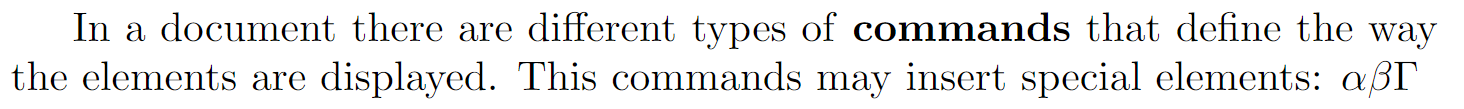In the previous example, there are different types of commands; for instance, \textbf will embolden the text passed as parameter to the command. In mathematical mode, there are special commands, such as \alpha, \beta and \Gamma to display Greek characters.

# Commands

Here is an example of LaTeX code with commands to create a bulleted list:

\documentclass{article}
\begin{document}
A list example:
\begin{itemize}
\item[\S] First item
\item Second item
\end{itemize}
\end{document}


This example produces the following output: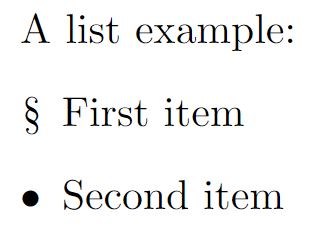The command \begin{itemize} starts an itemize environment—see the article about environments for more detail. Below the environment declaration is the command \item which tells LaTeX to start a new list entry: to format it, add a special mark (a small black dot called bullet) and indent the entry.

Some commands require one or more parameters; for example, the \textbf command (used above) which takes a single parameter—the text to show in bold type, which is written inside braces like this: \textbf{make this bold}.

There are also optional parameters which can be passed to a command to change its behaviour; those optional parameters have to be put inside brackets: [...]. In the example above, the command \item[\S] does the same as \item, except that inside the brackets is \S, which substitutes another character to replace the black dot before the text of the entry.

# Defining a new command

Although LaTeX is shipped with a huge number of commands it often becomes necessary to define your own special commands to simplify your work, reduce repetitive tasks or perform some complex formatting.

## Simple commands

New commands are defined by \newcommand; so let's see a simple example:

\documentclass{article}
\usepackage{amssymb}
\begin{document}
\newcommand{\R}{\mathbb{R}}
The set of real numbers are usually represented
by a blackboard bold capital R: $$\R$$.
\end{document}


This example produces the following output: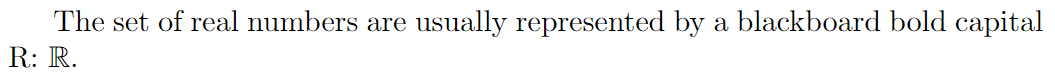The statement \newcommand{\R}{\mathbb{R}} has two parameters that define a new command, where:

• \R: is the name of the new command.
• \mathbb{R}: is what the new command does. In this case, the letter R will be written in blackboard boldface style. Use of the \mathbb command requires the amssymb package, which was added to the example above (in the preamble).

After the command is defined we can use it in the text, as demonstrated above. In this example, the new command is defined immediately before the paragraph in which it is used; however, scattering such definitions throughout your document is not considered best practice. Generally, definitions for new commands are collected together in the document preamble or, for larger collections, placed into a separate file that can be imported into your main document.

## Commands with parameters

It is also possible to create new commands that accept some parameters; for example:

\documentclass{article}
\usepackage{amssymb}
\begin{document}
\newcommand{\bb}{\mathbb{#1}}
Other numerical systems have similar notations.
The complex numbers $$\bb{C}$$, the rational
numbers $$\bb{Q}$$ and the integer numbers $$\bb{Z}$$.
\end{document}


This example produces the following output: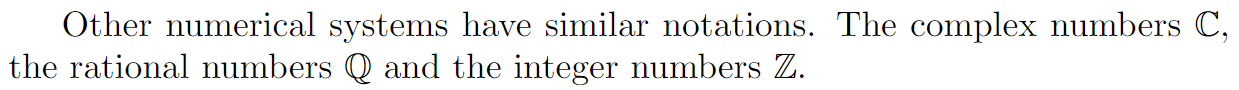The line \newcommand{\bb}{\mathbb{#1}} defines a new command that takes one parameter, where:

• \bb is the name of the new command.
•  is the number of parameters the new command will take.
• \mathbb{#1} is what the command actually does—its definition.

In this case, the parameter, referenced as #1, will be written using blackboard boldface characters. If a command needs more than one parameter, you can refer to each parameter using #1, #2 and so on. Up to 9 parameters are supported.

## Commands with optional parameters

User-defined commands can be made even more flexible than the examples shown so far: you can define commands that take optional parameters:

\documentclass{article}
\begin{document}

When writing many expressions with exponents we can simplify our task, and save time, by defining a suitable command:

\newcommand{\plusbinomial}{(#2 + #3)^#1}

We can use it like this: $\plusbinomial{x}{y}$

And even the exponent can be changed:

$\plusbinomial{a}{b}$
\end{document}


This example produces the following output: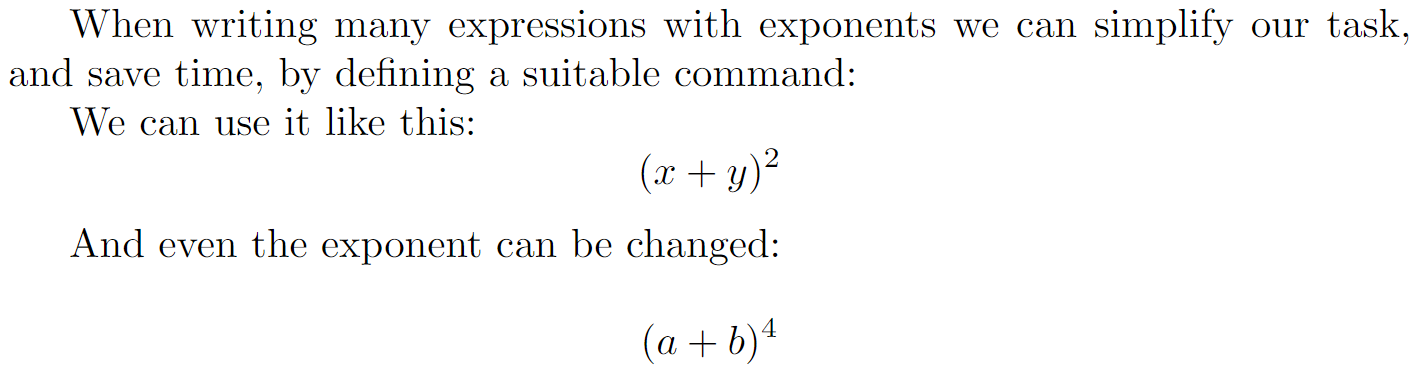Let's analyse the syntax of the line \newcommand{\plusbinomial}{(#2 + #3)^#1}:

• \plusbinomial is the name of the new command.
•  is the number of parameters the command will take, in this case 3.
•  is the default value for the first parameter. This is what makes the first parameter optional, if not passed it will use this default value.
• (#2 + #3)^#1 is what the command does. In this case it will put the second and third parameters in a "binomial format" to the power represented by the first parameter.

# Overwriting existing commands

If you try to define a new command which has the same name as an already existing LaTeX command then the compilation will fail with an error message. This is demonstrated in the following example which tries to define the (existing) \textbf command:

\documentclass{article}
\newcommand{\textbf}{#1}% This will not work
\begin{document}

\section{This will fail}
\end{document}


Compiling this example produces the following output: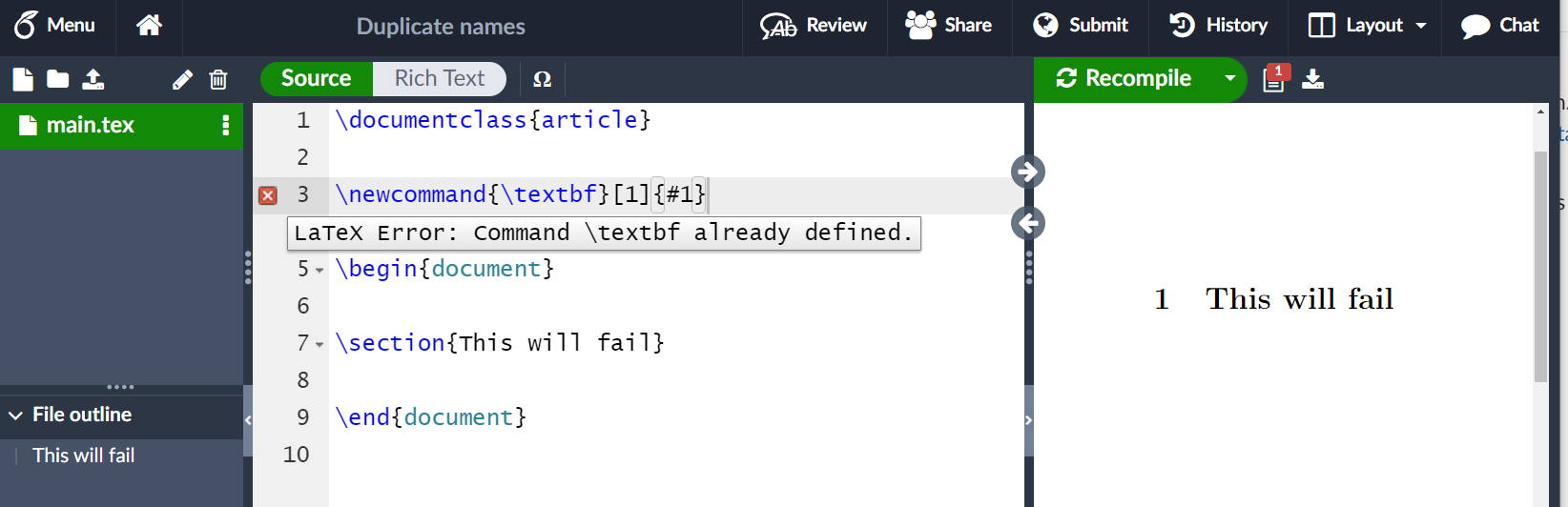As shown in the screen image, our attempt to define \textbf has failed: LaTeX generated the error message LaTeX Error: Command \textbf already defined.

If you really want to override an existing command this can be accomplished by \renewcommand, which uses the same syntax as \newcommand:

\documentclass{article}
\usepackage{amssymb}
\begin{document}

\renewcommand{\S}{\mathbb{S}}

The Riemann sphere (the complex numbers plus $\infty$) is
sometimes represented by $$\S$$.
\end{document}


This example produces the following output: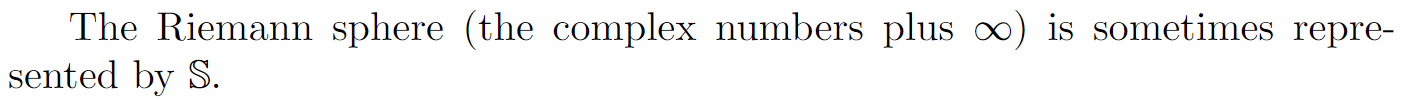In this example the command \S (see the example in the commands section) is overwritten to print a blackboard bold S.